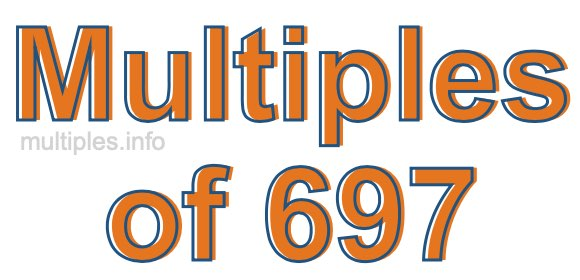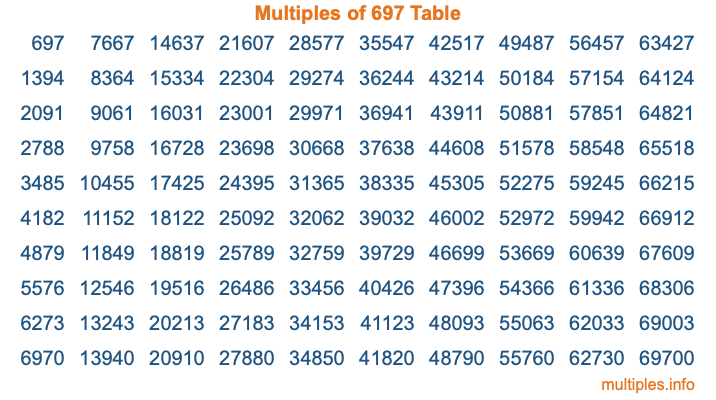Multiples of 697Welcome to the Multiples of 697 page. Here we will first teach you everything you will ever need to know about the multiples of 697, and then give you a study guide summary of everything we taught you to make sure you remember it all. Use this page to look up facts and learn information about the multiples of 697. This page will make you a multiples of six hundred ninety-seven expert!

Definition of Multiples of 697
Multiples of 697 are all the numbers that when divided by 697 equal an integer. Each of the multiples of 697 are called a multiple. A multiple of 697 is created by multiplying 697 by an integer.

Therefore, to create a list of multiples of 697, you start with 1 multiplied by 697, then 2 multiplied by 697, then 3 multiplied by 697, and so on for as long as you want. Thus, the list of the first five multiples of 697 is 697, 1394, 2091, 2788, and 3485. To see a larger list of multiples of 697, see the printable image of Multiples of 697 further down on this page. We also have a category where you can choose any nth multiple of 697.

Multiples of 697 Checker
The Multiples of 697 Checker below checks to see if any number of your choice is a multiple of 697. In other words, it checks to see if there is any number (integer) that when multiplied by 697 will equal your number. To do that, we divide your number by 697. If the the quotient is an integer, then your number is a multiple of 697.

Is  a multiple of 697?

Least Common Multiple of 697 and ...
A Least Common Multiple (LCM) is the lowest multiple that two or more numbers have in common. This is also called the smallest common multiple or lowest common multiple and is useful to know when you are adding our subtracting fractions. Enter one or more numbers below (697 is already entered) to find the LCM.

Check out our LCM Calculator if you need more details about the Least Common Multiple or if you need the LCM for different numbers for adding and subtraction fractions.

nth Multiple of 697
As we stated above, 697 is the first multiple of 697, 1394 is the second multiple of 697, 2091 is the third multiple of 697, and so on. Enter a number below to find the nth multiple of 697.

th multiple of 697

Multiples of 697 vs Factors of 697
697 is a multiple of 697 and a factor of 697, but that is where the similarities end. All postive multiples of 697 are 697 or greater than 697. All positive factors of 697 are 697 or less than 697.

Below is the beginning list of multiples of 697 and the factors of 697 so you can compare:

Multiples of 697: 697, 1394, 2091, 2788, 3485, etc.

Factors of 697: 1, 17, 41, 697

As you can see, the multiples of 697 are all the numbers that you can divide by 697 to get a whole number. The factors of 697, on the other hand, are all the whole numbers that you can multiply by another whole number to get 697.

It's also interesting to note that if a number (x) is a factor of 697, then 697 will also be a multiple of that number (x).

Multiples of 697 vs Divisors of 697
The divisors of 697 are all the integers that 697 can be divided by evenly. Below is a list of the divisors of 697.

Divisors of 697: 1, 17, 41, 697

The interesting thing to note here is that if you take any multiple of 697 and divide it by a divisor of 697, you will see that the quotient is an integer.

Multiples of 697 Table
Below is an image of the first 100 multiples of 697 in a table. The table is in chronological order, column by column. The first column has the first ten multiples of 697, the second column has the next ten multiples of 697, and so on.The Multiples of 697 Table is also referred to as the 697 Times Table or Times Table of 697. You are welcome to print out our table for your studies.

Negative Multiples of 697
Although not often discussed or needed in math, it is worth mentioning that you can make a list of negative multiples of 697 by multiplying 697 by -1, then by -2, then by -3, and so on, to get the following list of negative multiples of 697:

-697, -1394, -2091, -2788, -3485, etc.

Multiples of 697 Summary
Below is a summary of important Multiples of 697 facts that we have discussed on this page. To retain the knowledge on this page, we recommend that you read through the summary and explain to yourself or a study partner why they hold true.

There are an infinite number of multiples of 697.

A multiple of 697 divided by 697 will equal a whole number.

697 divided by a factor of 697 equals a divisor of 697.

The nth multiple of 697 is n times 697.

The largest factor of 697 is equal to the first positive multiple of 697.

697 is a multiple of every factor of 697.

697 is a multiple of 697.

A multiple of 697 divided by a divisor of 697 equals an integer.

697 divided by a divisor of 697 equals a factor of 697.

Any integer times 697 will equal a multiple of 697.

Multiples of a Number
Here you can get the multiples of another number, all with the same attention to detail as we did for multiples of 697 on this page.

Multiples of
Multiples of 698
Did you find our page about multiples of six hundred ninety-seven educational? Do you want more knowledge? Check out the multiples of the next number on our list!